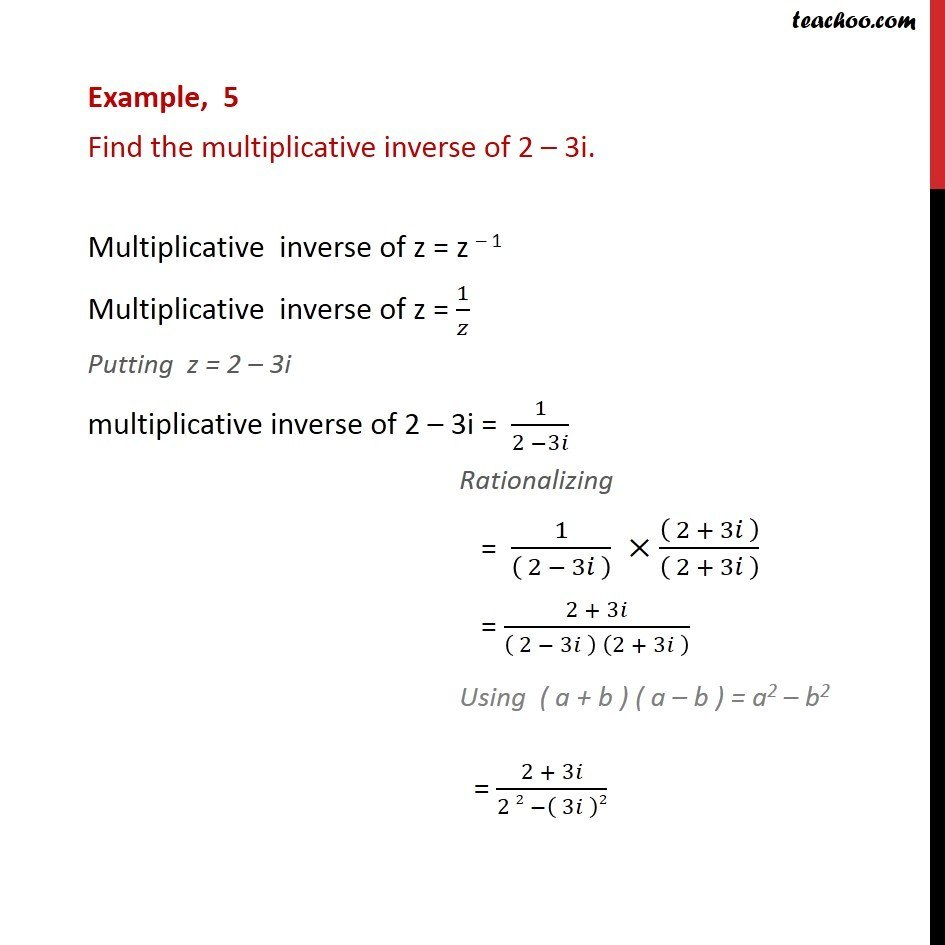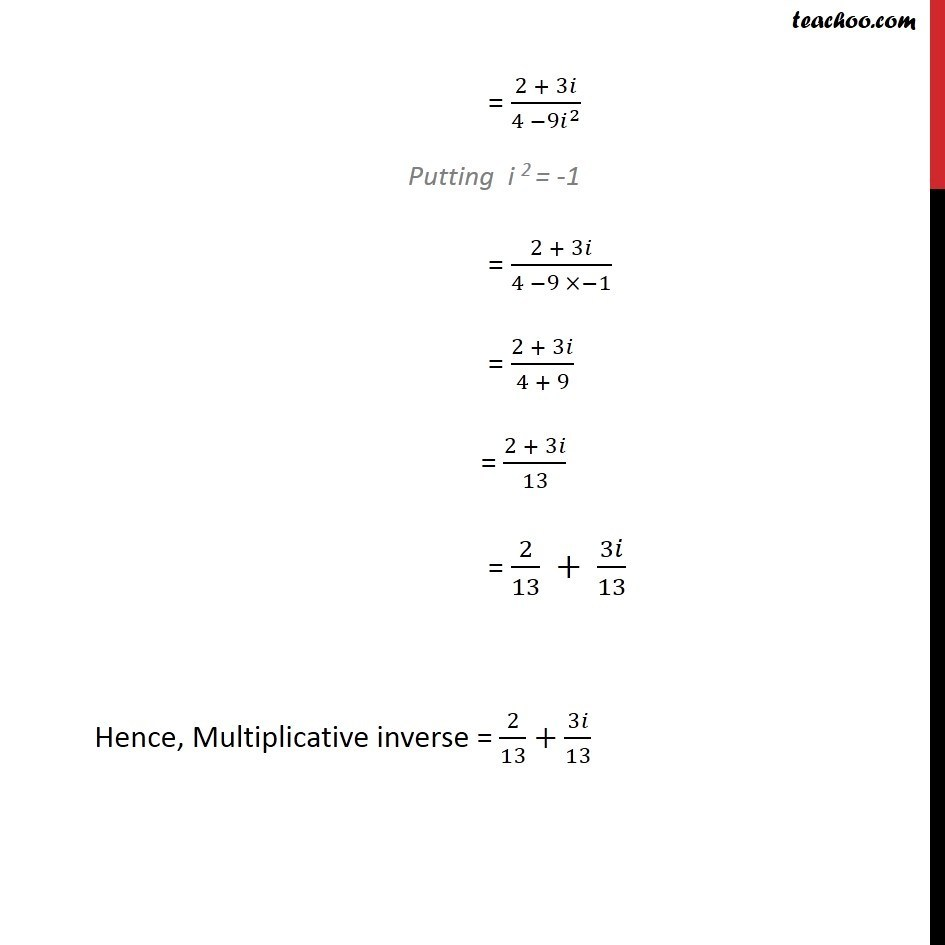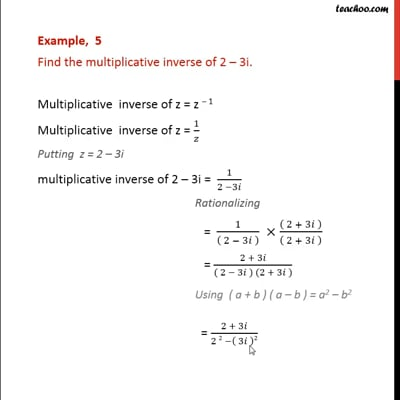Examples

Chapter 5 Class 11 Complex Numbers
Serial order wiseThis video is only available for Teachoo black users

Solve all your doubts with Teachoo Black (new monthly pack available now!)

### Transcript

Example, 5 Find the multiplicative inverse of 2 – 3i. Multiplicative inverse of z = z – 1 Multiplicative inverse of z = 1/𝑧 Putting z = 2 – 3i multiplicative inverse of 2 – 3i = 1/(2 −3𝑖) Rationalizing = 1/(( 2 − 3𝑖 ) ) ×(( 2 + 3𝑖 ))/(( 2 + 3𝑖 ) ) = (2 + 3𝑖)/(( 2 − 3𝑖 ) (2 + 3𝑖 ) ) Using ( a + b ) ( a – b ) = a2 – b2 = (2 + 3𝑖)/(2 2 −( 3𝑖 )2) = (2 + 3𝑖)/(4 −9𝑖^2 ) Putting i 2 = -1 = (2 + 3𝑖)/(4 −9 ×−1) = (2 + 3𝑖)/(4 + 9) = (2 + 3𝑖)/13 = 2/13 + 3𝑖/13 Hence, Multiplicative inverse = 2/13+3𝑖/13